Back to the Table of Contents

# Trigonometric Identities

## The Pythagorean Properties

a2 + b2 = c2                       Pythagorean Theorem (a and b are legs of a right triangle).
a2/c2+b2/c2 = 1                 Divide through by c2 (c is the hypotenuse).
sin2x + cos2x = 1               Since sin is opposite/hypotenuse and cos is adjacent/hypotenuse.
tan2x + 1 = sec2x               Divide through by cos2x—tan x = sin x/cos x (opposite/adjacent).
1 + cot2x = csc2x               Divide through instead by sin2x.

## The Reciprocal Properties

csc x = 1/sin x   and   sin x = 1/csc x               cosecant is the reciprocal of sine.
sec x = 1/cos x   and   cos x = 1/sec x             secant is the reciprocal of cosine.
cot x = 1/tan x     and   tan x = 1/cot x             cotangent is the reciprocal of tangent.
Remember, there is only one cofunction in a reciprocal pair.

## Which are Even, which are Odd.

Consider the even functions y = x0; y = x2; and y = x4. They are symmetric about the y-axis.
Whereas, the odd functions y = x1; and y = x3 are symmetric about the origin.
The two trigonometric functions cos x and its reciprocal sec x are even.
The other four trigonometric functions (sin x, csc x, tan x, and cot x) are all odd.
For even functions f(x) = f(-x), whereas for odd functions f(x) = -f(-x) or f(-x) = -f(x).

## Cofunction Properties

sin x=cos(90°-x)               sin and cos are both sinusoids, just displaced.
tan x=cot(90°-x)               tan and cot have a period of 180° (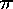) not 360°(2) like sin and cos.
sec x=csc(90°-x)               sec and csc have a period of 360°(2) just like sin and cos.

## Composite-Arguments

cos(A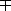B) = cosA cosB ± sinA sinB
sin(A ± B) = sinA cosB ± cosA sinB
tan(A ± B) = (tanA ± tanB) / (1tanA tanB)

## Double-Arguments

sin 2x = 2 sin x cos x
cos 2x = cos2x - sin2x = 1 - 2 sin2x = 2 cos2x - 1
tan 2x = 2 tan x /(1 - tan2x)
Note how these can also be derived from the Composite Argument formulae by letting A = B.
cos2x = (1 + cos 2x)/2
sin2x = (1 - cos 2x)/2
These last two are also known as and used as power reducing formulae.

## Half-Arguments

sin x/2 = ±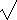((1 - cos x)/2)
cos x/2 = ±((1 + cos x)/2)
tan x/2 = ±((1 - cos x)/(1 + cosx)) = sin x/(1 + cos x) = (1 - cos x)/sin x

## Sum and Product

2cos Acos B = cos(A+B) + cos(A-B)
2sin Asin B = -cos(A+B) + cos(A-B)
2sin Acos B = sin(A+B) + sin(A-B)
2cos Asin B = sin(A+B) - sin(A-B)
cos A + cos B = 2cos0.5(A+B)cos0.5(A-B)
cos A - cos B = -2sin0.5(A+B)sin0.5(A-B)
sin A + sin B = 2sin0.5(A+B)cos0.5(A-B)
sin A - sin B = 2cos0.5(A+B)sin0.5(A-B)

## Linear Combination of Sine and Cosine

Acos x + Bsin x = Ccos(x-D); This is where C is the square root of A2 + B2 and cos(D) = A/C and sin(D) = B/C.

Hint: Draw a reference triangle. Calculate the angle. Determine which quadrant the angle is located by comparing the signs of A and B. If (A,B) is: (+,+) = I, (-,+) = II, (-,-) = III, and (+,-) = IV. Place those values into the formula above. An example of this would be: 2cos x - 2sin x. C2 = 4 + 4= 8, C = 2(2)½. The quadrant with a negative sinx and positve cosx is the fourth quadrant. Thus the expression simplifies to 2(2)½cos(x- 315°).

## Obtaining sec-1x, csc-1x, and cot-1x

The notation used for sin2x is confusing on two fronts. First, you can't enter it this way on most calculators. Rather, you must enter (sin x)2. Second, sin-1 is commonly used to indicate the inverse sine function or arcsine. Since we also commonly use the reciprocal functions of secant, cosecant, and cotangent, beginning students easily get confused to say the least.

If y = sin x, then 1/y = csc x. Thus x = sin-1y and x = csc-1 1/y. Similar reason can be used to derive the additional relationships below.

sec-1x=cos-11/x
csc-1x=sin-11/x
cot-1x=tan-11/x

Put here also info regarding principle value, domain restrictions, etc.

## The Sine, Cosine and Tangent of 15°

sin 15° = sin(45°-30°)                                     Forester 3.3#27-29, DWCF 7.4 Ex 1, #1-2
= sin 45°cos 30° - cos 45°sin 30°
= (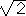/2) (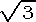/2) - (½)(/2)
= (6 -)/4

cos 15° = cos(45°-30°)
= cos 45°cos 30° + sin 45°sin 30°
= (/2) (/2) + (/2) (½)
= (6 +)/4)

tan 15° = tan(45°-30°)
= (tan 45° - tan 30°) / (1 + tan 45° tan 30°)
= (1 -/3) / (1 +/3)
= (3 -) / (3 +)                               multiply top and bottom by (3 -)
= (9 - 6+ 3) / (9 - 3)                         to make the denominator real.
= 2 -## The Derivation of Sin 18°

##### This is the solution to problem number 37 in section 3.5 of Foerster's Precalculus.

sin 72° = 2 sin 36° cos 36°                                 by the double angle relationship.
sin 72° = 4 sin 18° cos 18° (1 - 2sin2 18°)         by the double angle relationship, again.
cos 18° = 4 sin 18° cos 18° (1 - 2sin2 18°)         by the cofunction properties: sin 72° = cos 18°.
1 = 4 sin 18° (1 - 2sin2 18°)                       Let x = sin 18°, this is known as
1 = 4x(1-2x2)                                             substitution, a useful technique in calculus.
8x3-4x+1 = 0                                                         A product is zero only when one of its factors is zero.
8x3-4x+1 = (2x-1)(4x2+2x-1)=0                           (2x-1)=0 implies x= ½=sin 30° > sin 18° ;
Since we know sin is increasing on [0°,90°].
x = (-2 ±(4 + 4•4•1))/8                       So we must solve the other factor,
= (-2 ±20)/8                                     using the quadratic formula.
= (-2 ±45)/8
= (-1 ±5)/4                                       But the sin 18° > 0, so it cannot be negative.
sin 18°   = ((5)-1)/4                                         Hence the middle root is the one we want.

## The Derivation of Sin 1°

We now have most of what we need to prove the sin 1° to be an algebraic number. First we find the sin 3° by using the sines and cosines of 15° and 18° and the composite argument formula. Then we derive the formula for sin 3x=3sin x-4sin3x by repeated applications of the composite argument formula. The sin 1° can then be expressed as the root of this equation. (Note: "An algebraic number is a number x which satisfies an algebraic equation, i.e. an equation a0xn + a1xn-1 +...+an =0, where a0, a1,... are integers, not all zero. Any number defined by an equation as above, where a0, a1,..., an are algebraic, is algebraic. Hardy/Wright, An Introduction to the Theory of Numbers, 1979.) This was one of my favorite tests in Precalculus for 1993-1999—the whole test was a proof that sin 1° was algebraic.

BACK HOMEWORK ACTIVITY CONTINUE